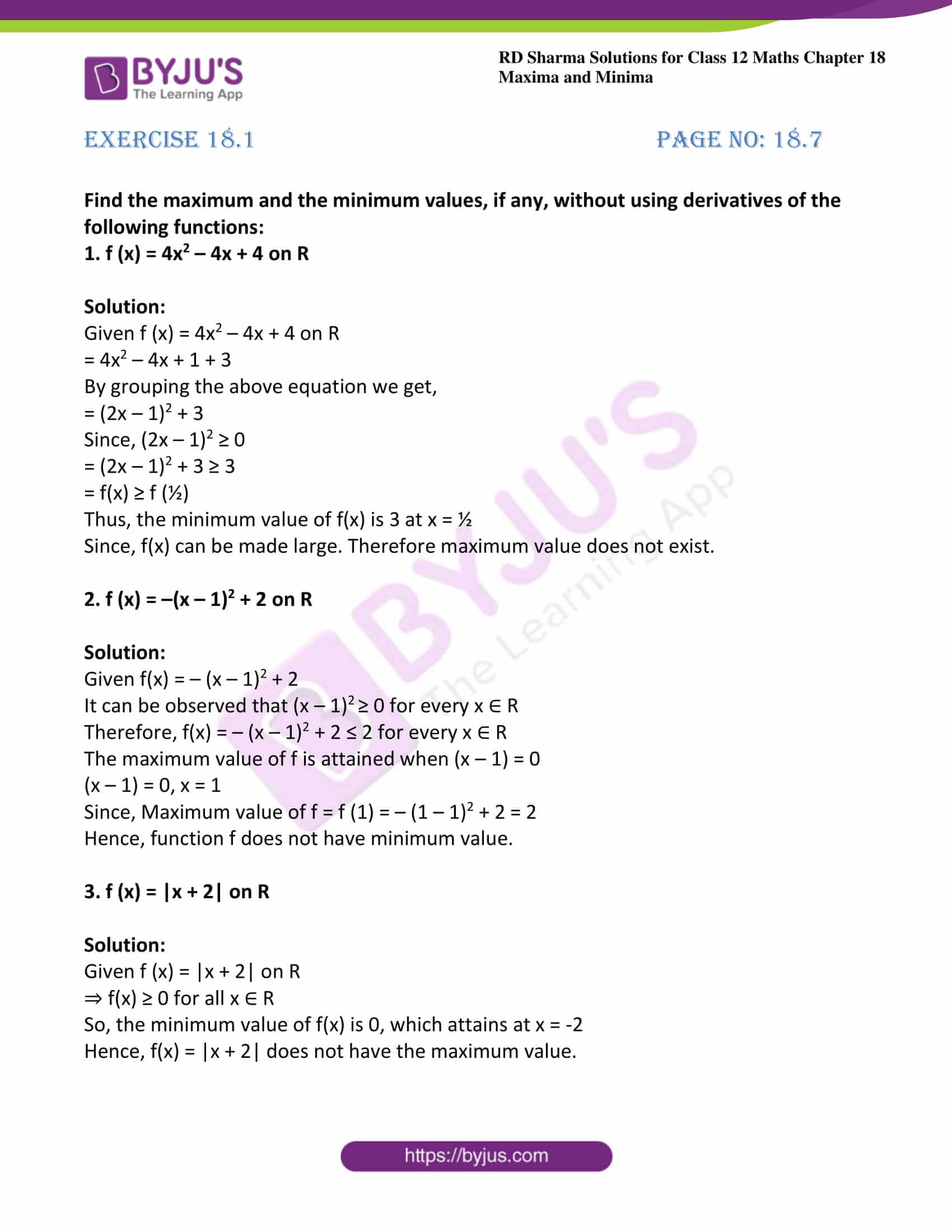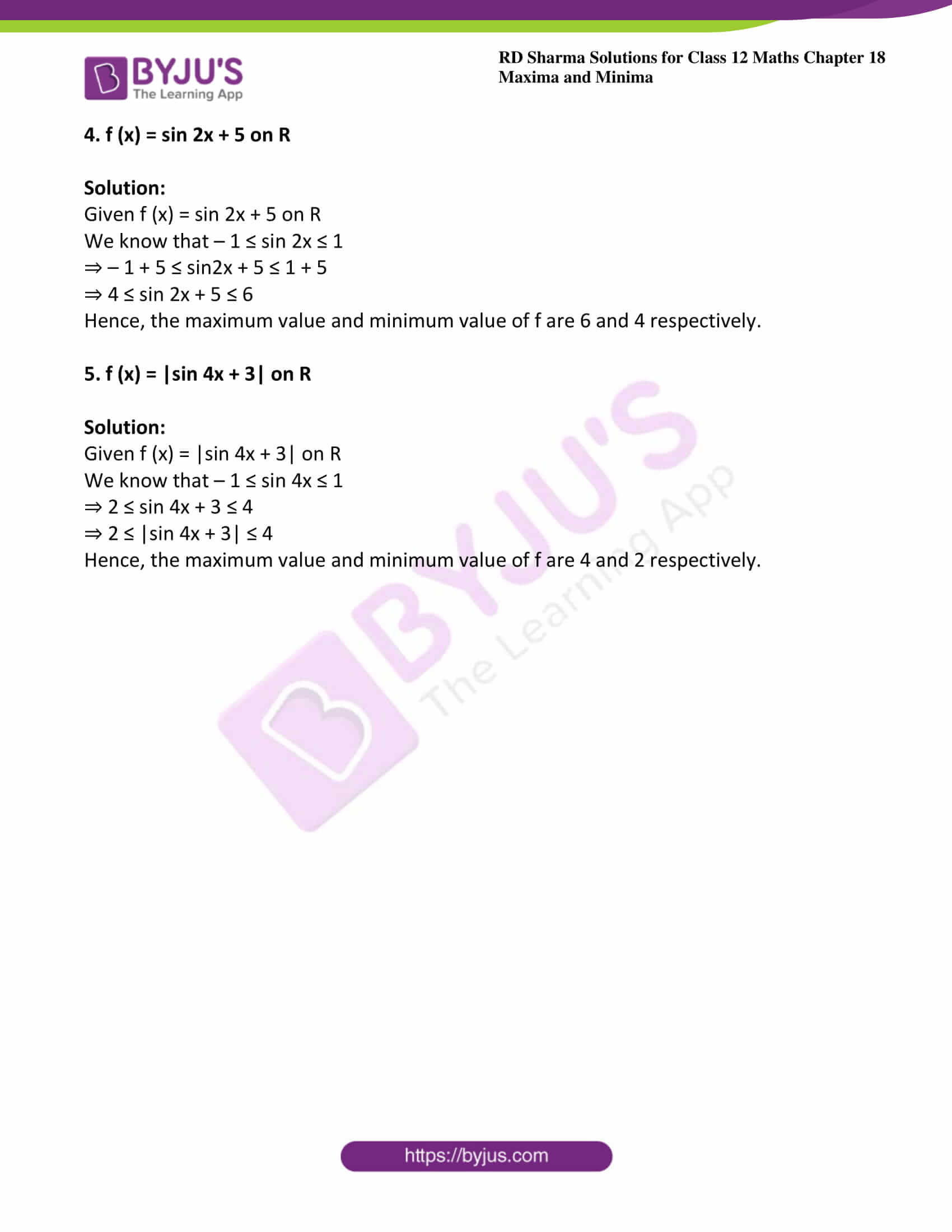# RD Sharma Solutions For Class 12 Maths Exercise 18.1 Chapter 18 Maxima and Minima

RD Sharma Solutions for Class 12 Maths Exercise 18.1 Chapter 18 Maxima and Minima are available here. Maximum and minimum values of a function in its domain are the main topics focused upon under this exercise. This exercise consists of two levels, according to the increasing order of difficulties.

By solving these levels, students will be thorough in crucial topics provided in the syllabus. The PDFs of RD Sharma Solutions for Class 12 Maths Exercise 18.1 of Chapter 18 Maxima and Minima are available here. Students can easily access and download the PDFs from the given links. Some of the important topics of this exercise are provided here.

• Maximum and minimum values of a function in its domain
• Definition and meaning of maximum
• Definition and meaning of minimum

## Download the PDF of RD Sharma Solutions For Class 12 Chapter 18 – Maxima and Minima Exercise 18.1### Access other exercises of RD Sharma Solutions For Class 12 Chapter 18 – Maxima and Minima

Exercise 18.2 Solutions

Exercise 18.3 Solutions

Exercise 18.4 Solutions

Exercise 18.5 Solutions

### Exercise 18.1 Page No: 18.7

Find the maximum and the minimum values, if any, without using derivatives of the following functions:

1. f (x) = 4x2 – 4x + 4 on R

Solution:

Given f (x) = 4x2 – 4x + 4 on R

= 4x2 – 4x + 1 + 3

By grouping the above equation we get,

= (2x – 1)2 + 3

Since, (2x – 1)2 ≥ 0

= (2x – 1)2 + 3 ≥ 3

= f(x) ≥ f (½)

Thus, the minimum value of f(x) is 3 at x = ½

Since, f(x) can be made large. Therefore maximum value does not exist.

2. f (x) = –(x – 1)2 + 2 on R

Solution:

Given f(x) = – (x – 1)2 + 2

It can be observed that (x – 1)2 ≥ 0 for every x ∈ R

Therefore, f(x) = – (x – 1)2 + 2 ≤ 2 for every x ∈ R

The maximum value of f is attained when (x – 1) = 0

(x – 1) = 0, x = 1

Since, Maximum value of f = f (1) = – (1 – 1)2 + 2 = 2

Hence, function f does not have minimum value.

3. f (x) = |x + 2| on R

Solution:

Given f (x) = |x + 2| on R

⇒ f(x) ≥ 0 for all x ∈ R

So, the minimum value of f(x) is 0, which attains at x = -2

Hence, f(x) = |x + 2| does not have the maximum value.

4. f (x) = sin 2x + 5 on R

Solution:

Given f (x) = sin 2x + 5 on R

We know that – 1 ≤ sin 2x ≤ 1

⇒ – 1 + 5 ≤ sin2x + 5 ≤ 1 + 5

⇒ 4 ≤ sin 2x + 5 ≤ 6

Hence, the maximum value and minimum value of f are 6 and 4 respectively.

5. f (x) = |sin 4x + 3| on R

Solution:

Given f (x) = |sin 4x + 3| on R

We know that – 1 ≤ sin 4x ≤ 1

⇒ 2 ≤ sin 4x + 3 ≤ 4

⇒ 2 ≤ |sin 4x + 3| ≤ 4

Hence, the maximum value and minimum value of f are 4 and 2 respectively.